Next: Systematic errors Up: Evaluation of uncertainty: general Previous: Direct measurement in the   Contents

## Indirect measurements

The case of quantities measured indirectly is conceptually very easy, as there is nothing to think'. Since all values of the quantities are associated with random numbers, the uncertainty on the input quantities is propagated to that of output quantities, making use of the rules of probability. Calling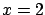,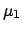and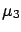the generic quantities, the inferential scheme is: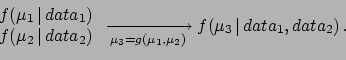(2.4)

The problem of going from the probability density functions (p.d.f.'s) ofandto that ofmakes use of probability calculus, which can become difficult, or impossible to do analytically, if p.d.f.'s or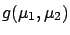are complicated mathematical functions. Anyhow, it is interesting to note that the solution to the problem is, indeed, simple, at least in principle. In fact,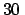is given, in the most general case, by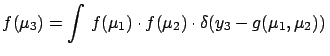dd(2.5)

where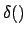is the Dirac delta function. The formula can be easily extended to many variables, or even correlations can be taken into account (one needs only to replace the product of individual p.d.f.'s by a joint p.d.f.). Equation () has a simple intuitive interpretation: the infinitesimal probability elementdepends on how many' (we are dealing with infinities!) elements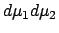contribute to it, each weighed with the p.d.f. calculated in the point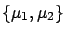. An alternative interpretation of Eq. (), very useful in applications, is to think of a Monte Carlo simulation, where all possible values ofandenter with their distributions, and correlations are properly taken into account. The histogram ofcalculated fromwill `tend' tofor a large number of generated events.2.16

In routine cases the propagation is done in an approximate way, assuming linearization ofand normal distribution of. Therefore only variances and covariances need to be calculated. The well-known error propagation formulae are recovered (Section), but now with a well-defined probabilistic meaning.Next: Systematic errors Up: Evaluation of uncertainty: general Previous: Direct measurement in the   Contents
Giulio D'Agostini 2003-05-15